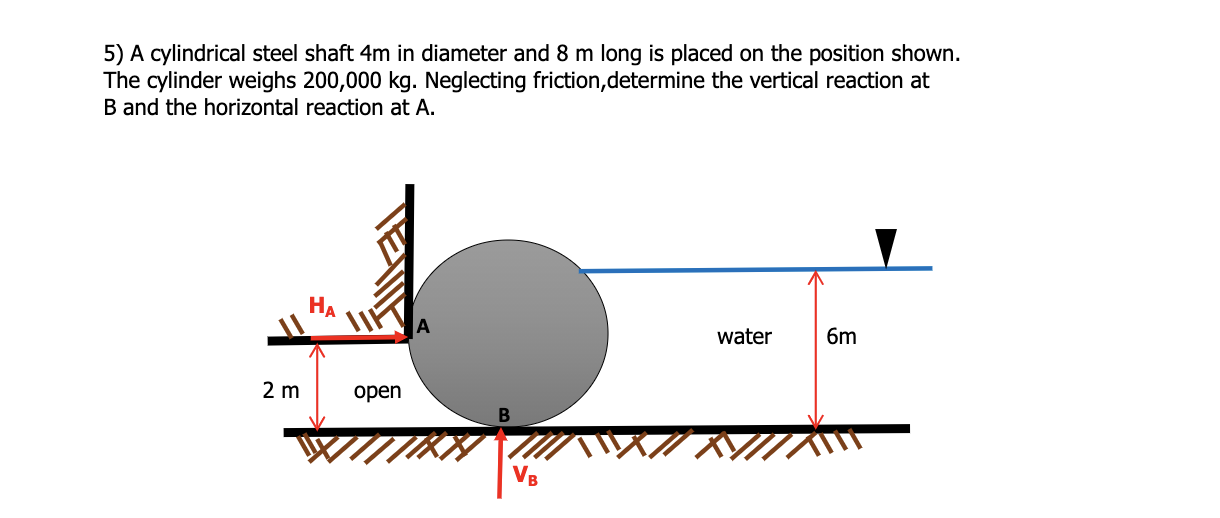# A cylindrical steel shaft 4m in diameter and 8 m long is placed on the position shown. The cylinder weighs 200,000 kg. Neglecting friction, determine the vertical reaction at B and the horizontal reaction at A.

Question-AnswerCategory: Fluid MechanicsA cylindrical steel shaft 4m in diameter and 8 m long is placed on the position shown. The cylinder weighs 200,000 kg. Neglecting friction, determine the vertical reaction at B and the horizontal reaction at A.

A cylindrical steel shaft 4m in diameter and 8 m long is placed on the position shown. The cylinder weighs 200,000 kg. Neglecting friction, determine the vertical reaction at B and the horizontal reaction at A.Sol: Information provided in the question:
Diameter of steel shaft (D) = 4 m
Length of steel shaft (L) = 8 m
Total weight of the cylinder (Wc) = 200,000 Kg
=> First we calculate the total vertical reaction at Point (B) : Vb
As we can see the cylinder has displaced some water from the Right side so due to the displacement of water, it will exert an equal amount of vertical force in the upward direction (Fv) but there is no water on left side so the force is exerted on it.
=> Also there is downward force acting on cylinder due to self weght = FwHence final condition of vertical force
=>Vertical Reaction at B:
Vb = Fv – Fw
= Density of water x Volume of cylinder – Wc x 9.81
= 9.810 x (pi / 4) x D^2 x L – (200000 x 9.81)
= 985.708 – 1962
= 976.292 KN { Downward direction}
=> Now the net horizontal reaction at Point A:=> Hence the horizontal reaction at A is given by:
Ha = Fh = Area of stress block of water x Length of cylinder
= 0.5 x (Density of water x 6) x 6 x 8
= 0.5 x 9.81 x 6x6x8
= 1412.64 KN
=> So,
Ha = 1412.64 KN
Vb = 976.292 KN